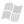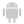ONLINE HELP WINDEV, WEBDEV AND WINDEV MOBILEVersion: 151617181920212223242526
 Home |Sign in | EnglishHelpWLanguageWLanguage functionsControls, pages and windowsTable functionsPrefix syntaxFormulaAddPresentationExampleConditions for adding the row containing the custom calculation formulaRecalculating dataCustomizing the calculation rowSee alsoAddAddColumnAddInProgressAddLineAddLineWithAnimationAddTitleHeaderAdjustBreakSubscriptCellSelectCellSelectOccurrenceColumnSubscriptCountDeleteDeleteAllDeleteSelectDeleteWithAnimationDisableFilterDisplayEndEndFillingEnumBreakEnumColumnFilteredColumnFixColumnFormulaAddFormulaDeleteAllInfoXYInputInProgressInputSearchInsertInsertColumnInsertLineInsertLineWithAnimationModifyModifyColumnModifyLineMoveColumnMoveLinePositionPrintRecNumRefreshVisibleRestorePositionAndSelectionSaveSavePositionAndSelectionSelectSelectCountSelectMinusSelectPlusSelectToFileSetFocusSortSortedColumnStartFillingSwapLineFunctions for managing Table controls (prefixed syntax)
 Conditions for adding the row containing the custom calculation formulaRecalculating dataCustomizing the calculation row WINDEVWEBDEVWINDEV MobileOthersAdds a calculated row into a Table control by providing the procedures for custom calculations.Example TABLE_MyTable.FormulaDeleteAll()nRow is intnRow = TABLE_MyTable.COL_Num.FormulaAdd("Positive mean", ProcInit, ProcAdd, ProcEnd)// Change the background color of the row for custom calculationCOL_Num[nRow]..BackgroundColor = LightRed nCounter is intINTERNAL PROCEDURE ProcInit() nCounter = 0 RESULT 0END INTERNAL PROCEDURE ProcAdd(Accumulator, ColValue) // Ignores the negative numbers or NULL IF (ColValue <= 0) RESULT Accumulator nCounter++ RESULT Accumulator + ColValueENDINTERNAL PROCEDURE ProcEnd(Accumulator) IF nCounter = 0 THEN RESULT 0 // Calculate the mean RESULT Accumulator/nCounterEND nRow2 is intnRow2 = TABLE_TableControl.COL_Time.FormulaAdd("Average duration", Null, ... AverageDuration_Iteration, AverageDuration_End) INTERNAL PROCEDURE AverageDuration_Iteration(Accumulator, ColValue) RESULT Accumulator + [TAB] + ColValueEND INTERNAL PROCEDURE AverageDuration_End(Accumulator) nSum is 8-byte int nNbValid is int FOR EACH STRING sValue OF Accumulator SEPARATED BY TAB IF ValidTime(sValue) THEN nSum += TimeToInteger(sValue) nNbValid++ END END RESULT TimeToString(IntegerToTime(nSum/nNbValid), "HH:MM:SS")END Syntax = .FormulaAdd( , , , ) : IntegerSubscript of the row containing the formula. : Control nameName of column into which the formula will be added. : Character string (with quotes)Caption of the additional row where the calculation will be displayed. If this caption does not exist, the row will be created. If this caption exists but not for the specified column, the calculation is displayed in the existing row but for the specified column. If this caption exists for the specified column, a WLanguage error occurs. : Character string (with or without quotes)Name of WLanguage procedure ("callback" procedure) that will be called to initialize the formula. This procedure has the following format: PROCEDURE ()// your codeRESULT where is the value to return for the first iteration of the calculation.NULL if the formula requires no initialization process. : Character string (with or without quotes)Name of WLanguage procedure ("callback" procedure) that will be called for each iteration of the formula (each table row). This procedure has the following format: PROCEDURE (, )// Called for each table row// Your codeRESULT where: is the value coming from the previous calculation (initialization or previous iteration). is the value of the current column used to calculate this iteration. is the new value to return for the next iteration or at the end of calculation.NULL if the formula requires no initialization process. : Character string (with or without quotes)Name of the WLanguage procedure ("callback" procedure) that will be called to end the formula. This procedure has the following format: PROCEDURE ()// your codeRESULT where is the value to return corresponding to the value of end of calculation.NULL if the formula requires no ending process.Remarks Conditions for adding the row containing the custom calculation formulaIf the calculation named does not exist in the table, a new calculation row is added below the table (after the existing calculations). If a calculation named was already defined for another column, the calculation is displayed for the specified column in the existing calculation row.If a calculation named was already defined for the same column, a WLanguage error occurs.Only 5 custom calculation rows can be added. Remark: This function can be used: on the columns found in a Table or TreeView Table control. on the columns found in the memory or browsing controls.Recalculating dataThe custom calculation rows are automatically recalculated as soon as the content of the Table control changes.Tip: The iteration procedure is called for each row: we advise you not to perform slow calculations (avoid the accesses to the database for example).Customizing the calculation rowThe following syntax is used to customize the added row (caption, color, font, height, ...): [Row number].. = where can correspond to one of the properties that can be used on a column of a Table control. See Programming custom calculations in Table controls for more details.Component: wd260obj.dll Minimum version required Version 23 This page is also available for…
 Comments
 Click [Add] to post a comment
 Last update: 03/17/2021Send a report | Local help
 Registered trademarks. Copyright ©2009-2021 PC SOFT. All rights reservedThis website has been developed with WEBDEV and HFSQLWEBDEV, WINDEV Mobile and WINDEV programming